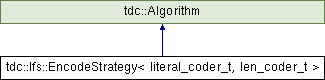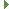tudocomp – The TU Dortmund Compression Framework
tdc::lfs::EncodeStrategy< literal_coder_t, len_coder_t > Class Template Reference

`#include <EncodeStrategy.hpp>`

Inheritance diagram for tdc::lfs::EncodeStrategy< literal_coder_t, len_coder_t >:## Public Member Functions

void encode (io::InputView &in, Output &output, rules &dictionary, non_terminal_symbols &nts_symbols)

void decode (Input &input, Output &output)Public Member Functions inherited from tdc::Algorithm
virtual ~Algorithm ()=default

Algorithm (Algorithm const &)=default

Algorithm (Algorithm &&)=default

Algorithmoperator= (Algorithm const &)=default

Algorithmoperator= (Algorithm &&)=default

Algorithm (Env &&env)
Instantiates an algorithm in the specified environment. More...

Envenv ()

const Envenv () const

## Static Public Member Functions

static Meta meta ()

## Detailed Description

### template<typename literal_coder_t, typename len_coder_t> class tdc::lfs::EncodeStrategy< literal_coder_t, len_coder_t >

Definition at line 42 of file EncodeStrategy.hpp.

## ◆ decode()

template<typename literal_coder_t , typename len_coder_t >
 void tdc::lfs::EncodeStrategy< literal_coder_t, len_coder_t >::decode ( Input & input, Output & output )
inline

Definition at line 190 of file EncodeStrategy.hpp.

## ◆ encode()

template<typename literal_coder_t , typename len_coder_t >
 void tdc::lfs::EncodeStrategy< literal_coder_t, len_coder_t >::encode ( io::InputView & in, Output & output, rules & dictionary, non_terminal_symbols & nts_symbols )
inline

Definition at line 62 of file EncodeStrategy.hpp.

## ◆ meta()

template<typename literal_coder_t , typename len_coder_t >
 static Meta tdc::lfs::EncodeStrategy< literal_coder_t, len_coder_t >::meta ( )
inlinestatic

Definition at line 54 of file EncodeStrategy.hpp.

The documentation for this class was generated from the following file: